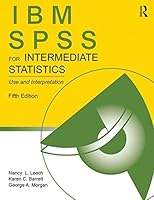# SPSS for Introductory and Intermediate Statistics, 5th Edition## Book Description

Designed to help readers analyze and interpret research data using SPSS, this user-friendly book shows readers how to choose the appropriate statistic based on the ; perform intermediate statistics, including multivariate statistics; interpret output; and write about the results. The book reviews research designs and how to assess the accuracy and reliability of data; how to determine whether data meet the assumptions of statistical tests; how to calculate and interpret effect sizes for intermediate statistics, including odds ratios for logistic analysis; how to compute and interpret post-hoc power; and an overview of basic statistics for those who need a review. Unique chapters on multilevel linear ; multivariate analysis of variance (MANOVA); assessing reliability of data; multiple imputation; mediation, moderation, and canonical correlation; and factor analysis are provided. SPSS syntax with output is included for those who prefer this format.

The new edition features:

• IBM SPSS version 22; although the book can be used with most older and newer versions
• New discusiion of intraclass correlations (Ch. 3)
• Expanded discussion of effect sizes that includes confidence intervals of effect sizes (ch.5)
• New on part and partial correlations and how they are interpreted and a new discussion on backward elimination, another useful multiple regression method (Ch. 6)
• New chapter on how to use a variable as a mediator or a moderator (ch. 7)
• Revised chapter on multilevel and hierarchical linear modeling (ch. 12)
• A new chapter (ch. 13) on multiple imputation that demonstrates how to deal with missing data
• Updated web resources for instructors including slides and answers to interpretation questions and extra problems and for students, data sets, chapter outlines, and study guides.

IBM SPSS for Intermediate Statistics, Fifth Edition

• all of the key SPSS windows needed to perform the analyses
• outputs with call-out boxes to highlight key points
• interpretation sections and questions to help students better understand and interpret the output
• extra problems with realistic data sets for practice using intermediate statistics
• Appendices on how to get started with SPSS, write research questions, and basic statistics.

An ideal supplement for courses in either intermediate/advanced statistics or research methods taught in departments of psychology, , and other social, behavioral, and health sciences. This book is also appreciated by researchers in these areas looking for a handy reference for SPSS

Chapter 1 Introduction
Chapter 2 Data Coding And Exploratory Analysis (Eda)
Chapter 3 Several Measures Of Reliability
Chapter 4 Exploratory Factor Analysis And Principal Components Analysis
Chapter 5 Selecting And Interpreting Inferential Statistics
Chapter 6 Multiple Regression
Chapter 7 Mediation, Moderation, And Canonical Correlation
Chapter 8 Logistic Regression And Discriminant Analysis
Chapter 9 Factorial Anova And Ancova
Chapter 10 Repeated-Measures And Mixed Anovas
Chapter 11 Multivariate Analysis Of Variance (Manova)
Chapter 12 Multilevel Linear Modeling/Hierarchical Linear Modeling
Chapter 13 Missing Data And Multiple Imputation